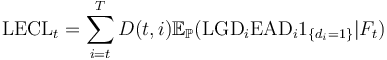## Definition

Lifetime Expected Credit Losses are the expected credit losses that result from all possible default events over the expected life of a financial instrument.

## Formula

Conceptually the definition is captured in the following mathematical expression$\mbox{LECL}_t = \sum_{i=t}^{T} D(t,i) \mathbb{E}_{\mathbb{P}} (\mbox{LGD}_{i} \mbox{EAD}_{i} 1_{ \{ d_{i} = 1 \} } | F_t )$
• Where t is the reporting date
• T is the Expected Life of the instrument
• i denotes possible times of default / loss (normally associated with instrument cashflows)
• di is the random (unknown) event of default at time i
• LGD denotes Loss Given Default
• EAD denotes Exposure at Default
• D(t,i) denotes the discount rate at time t (based on the Effective Interest Rate, for the different cashflow maturities i
• Ft denotes the subjective but Forward-Looking Information set used formulate the estimate at time t
• P denotes the subjective assignment of default probabilities to the events di

This formula captures the essence of the definition. In practice evaluation of LECL may utilize a variety of simplified / approximate forms. A common simplification is the use of the concept of Lifetime PD